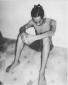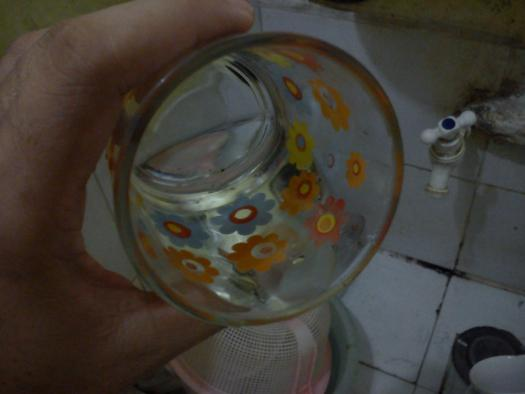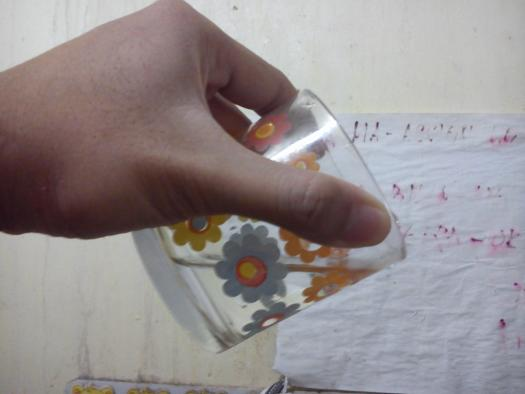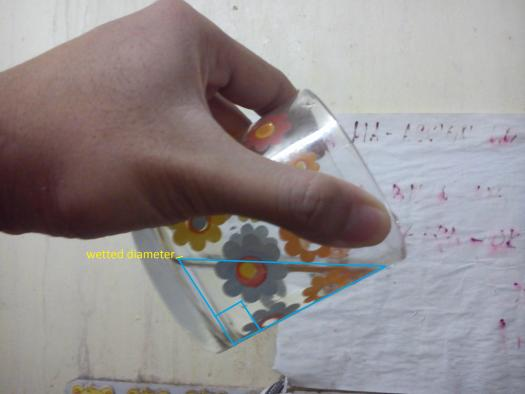# From a cylindrical jar 4 in. high and 6 in. in diameter, water is poured by tilting the jar until the center of the bottom is at

2 posts / 0 new
Renz Kolin Gaba...From a cylindrical jar 4 in. high and 6 in. in diameter, water is poured by tilting the jar until the center of the bottom is at
fitzmerl duron$\mathbf{For \space the \space first \space question:}$

We need a picture to visualize the problem.Looking deeper at the picture, we found out that the section made by a plane running parallel through the cylinder axis and wetted diameter is a:$$\color{blue}{\mathbf{Parabolic \space Segment}}$$

Well...it does look like an ellipse broke into two equal parts though...

$\mathbf{For \space the \space second \space question:}$

We need a picture to visualize the problem.Looking deeper at the picture, we found out that the section made by a plane running perpendicular through the wetted diameter is a:$$\color{blue}{\mathbf{Right \space Triangle}}$$

Alternate solutions are encouraged....

Subscribe to MATHalino on